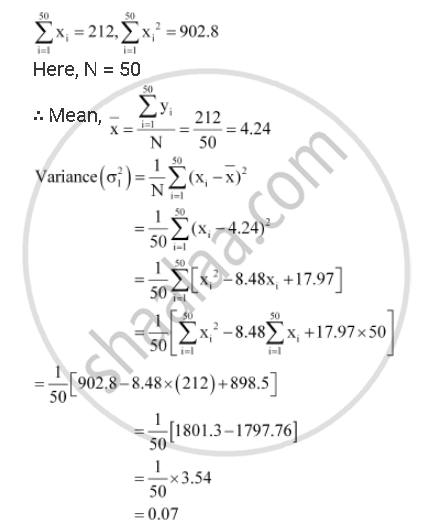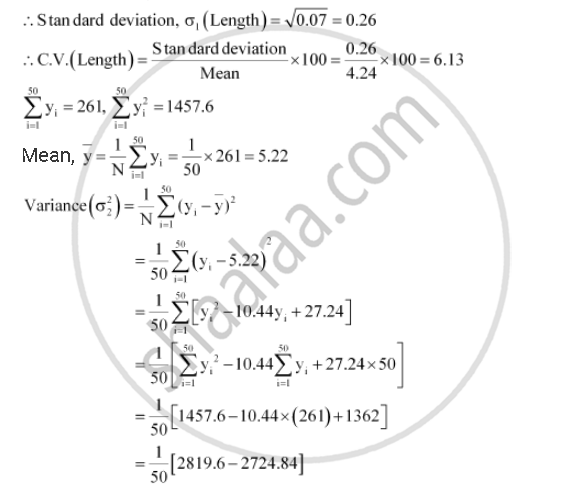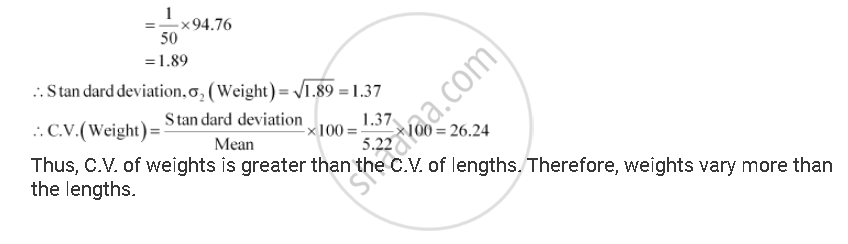CBSE (Arts) Class 11CBSE
Share

# The Sum and Sum of Squares Corresponding to Length X (In Cm) and Weight Y (In Gm) of 50 Plant Products Are Given Below: - CBSE (Arts) Class 11 - Mathematics

ConceptVariance and Standard Deviation Standard Deviation

#### Question

The sum and sum of squares corresponding to length (in cm) and weight (in gm) of 50 plant products are given below:

sum_(i-1)^50 x_i = 212, sum_(i=1)^50 x_i^2 = 902.8, sum_(i=1)^50 y_i = 261, sum_(i = 1)^50 y_i^2 = 1457.6

Which is more varying, the length or weight?

#### SolutionIs there an error in this question or solution?

#### APPEARS IN

Solution The Sum and Sum of Squares Corresponding to Length X (In Cm) and Weight Y (In Gm) of 50 Plant Products Are Given Below: Concept: Variance and Standard Deviation - Standard Deviation.
S